## Wednesday, April 27, 2011

### Random sampling script (C++)

Computers as machines with deterministic behavior cannot generate truly random numbers. However, they can be attached to external devices that can measure the random-like events from the physical processes (for example, the radio measuring atmospheric noise after the thunderstorm). Such devices, termed hardware random number generators, may be relatively slow in some cases, and they may produce a biased sequence of numbers (since some values could be more common, depending on the nature of the observed physical process). Computer algorithms, on the other side, can generate relatively quickly a sequence of pseudo-random numbers using a predefined mathematical formula (pseudo-random number generators or PRNGs). Since such numbers are made by the formula and thus could be predicted, they should be avoided for creating passwords or for any other cryptographic application. In biology studies, the predictability of PRNGs is not of a main concern. The pseudo-random number generators are commonly used for different purposes in biology. Randomization, for example, is used to generate random nucleotide or amino-acid sequences or shuffle the existing sequences for different test purposes. Features that should be controlled properly in PRNGs and that are of the most importance in biology studies are the uniform distribution of chosen values and the absence of short cycles of repetitions of randomly chosen numbers. As here is shown, in some instances the uniform deviates and the short cycling of random values may be mutually exclusive. However, there is a way to keep both of these essential characteristics of PRNGs when creating a generator for its successful implementation in biological data analysis.

Pseudorandom number generators are often based on linear congruential generators (LCGs). The following relation defines LCGs:

Xn+1 = (aXn + c) mod m

with X being a sequence of pseudorandom values, and m (modulus) defining the range of these values. Built-in rand() functions of various programming languages are based on LCGs that have a modulus equal to 21515 – 1 = 32,767 (e.g. in Microsoft Visual C++ that was used is this study). Thus, the range of most of the LCGs commonly used will be limited to the value of 2.

Defining a new range of random values, whether extending or contracting the initial range, might be done in many different ways. One of the algorithms often used in C++ for defining the desired (smaller) range of random numbers (RANGE_MAX) is based on the division of rand() with the value that equals RANGE_MAX +1. The remainder of division of rand() and RANGE_MAX +1 is considered a random number that is ranging from 0 to RANGE_MAX.

However, this algorithm is using the lower-order bits of random numbers, which exhibit much shorter cyclic behavior and are thus considered less “random”. This type of algorithms generally should be avoided. To test the cycling properties of this algorithm one could use the following code that is creating 262,144 random numbers ranging from 0-1.

// Algorithm type 1

#include <iostream>
#include <ctime>
#include <cstdlib>

using namespace std;

int main()
{
srand((unsigned)time(0));
long int i=0;
int random_integer;
while(i<262144)
{
random_integer = rand()%2;
cout<< random_integer <<endl;i++;
}
}

Obtained values were then translated to a 512x512 matrix and the matrix was plotted as an image of 512x512 pixels. Each pixel can be either in white or red color, representing values 0 and 1, respectively. The image is showing complex patterned profile, which is based on the shorter cycles of repetitions of chosen numbers (Figure 1). In addition, if rand()%4 is used to obtain random numbers in the range 0-3, the 512x512 pixel image revealed the similar patterned profile (Figure 2). Thus, such kinds of algorithms generally should be avoided because of the highly repetitive nature of their output.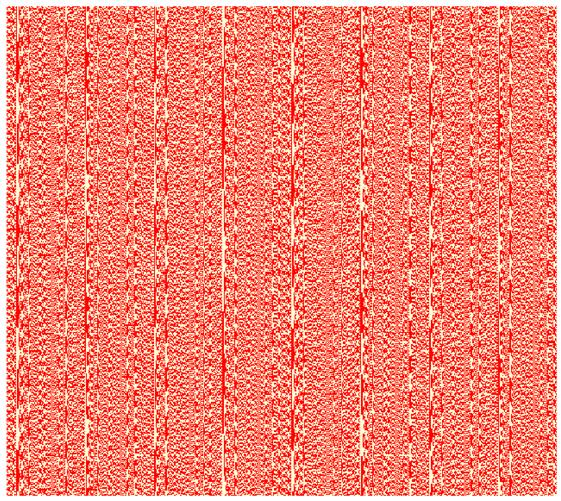Figure 1.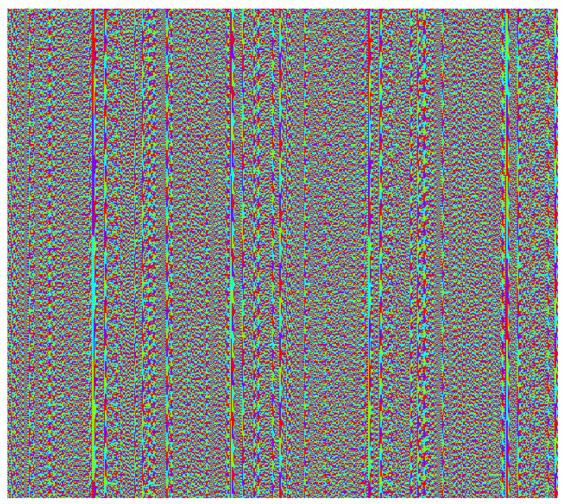Figure 2.

One of the algorithms that could be used to extend the range of random values in C++ is shown below. The following algorithm will generate 20 random numbers in a certain range (1-10 in this example).

// Algorithm type 2

#include <iostream>
#include <ctime>
#include <cstdlib>

using namespace std;
int main()
{
srand((unsigned)time(0));
int random_integer;
int lowest=1, highest=10;
int range=(highest-lowest)+1;
for(int index=0; index<20; index++)
{
random_integer = lowest+int(range*rand()/(RAND_MAX + 1.0));
cout << random_integer << endl;
}
}

However, the random numbers made by this algorithm are not following the uniform distribution. It could be calculated that the frequencies of numbers from 1-10 chosen by this algorithm are not the same, although the difference could not be seen by eye. Ratio rand()/(RAND_MAX +1) is an increment of 1/(RAND_MAX +1) = 1/32768 = 0.000030517578125, thus the number (range*rand()/(RAND_MAX + 1.0)) will be an increment of 10×0.000030517578125= 0.00030517578125. It could be calculated that out of 32768 possible increments, int function will round up:

0 in 3277 cases,
1 in 3277 cases,
2 in 3277 cases,
3 in 3277 cases,
4 in 3276 cases,
5 in 3277 cases,
6 in 3277 cases,
7 in 3277 cases,
8 in 3277 cases,
9 in 3276 cases.

Therefore, the probability of choosing the numbers 4 and 9 is lower than of the other numbers, and the distribution of chosen values will not be uniform. This may not be a problem for smaller ranges like this one, but in the cases of large ranges, this may raise serious issues, since the differences in frequencies become more significant. In the case of a range from 1-10,000, random numbers will be an increment of 0.30517578125 and thus they may occur either 3 or 4 times. In the case of the range of values from 1-1,000,000, random number will be generated as an increment of 30.517578125, thus the majority of numbers will never be chosen at all. To test whether algorithm type 2 exhibits such huge distribution defects, we selected 10,000,000 random numbers using a range of numbers from 1-1,000,000. Then we plotted their distribution in a much smaller window from 0-300 using the bin size of 1 (Figure 3). As it can be seen, only certain numbers may be chosen, while the rest will never be selected.Figure 3.

The use of this algorithm, thus, is extremely limited concerning our needs to extend the range and keep the uniform distribution of values. However, this algorithm is using also the higher-order bits of generated random numbers and thus it avoids short cycles of repetition, unlike the previous algorithm.

The same test of creating 262,144 random numbers in the range 0-1 gave a distribution that looks deprived from the patterns that were seen in the previous case (Figure 4). Similarly, in the case of the range 0-3 the image remains devoid of patterns (Figure 5).

// Algorithm type 2, example 2

#include <iostream>
#include <ctime>
#include <cstdlib>

using namespace std;

int main()
{
srand((unsigned)time(0));
long int i=0;
int random_integer;
while(i<262144)
{
random_integer = (int)(((double)rand()/((double)RAND_MAX+(double)1))*2);
cout<< random_integer <<endl;i++;
}
}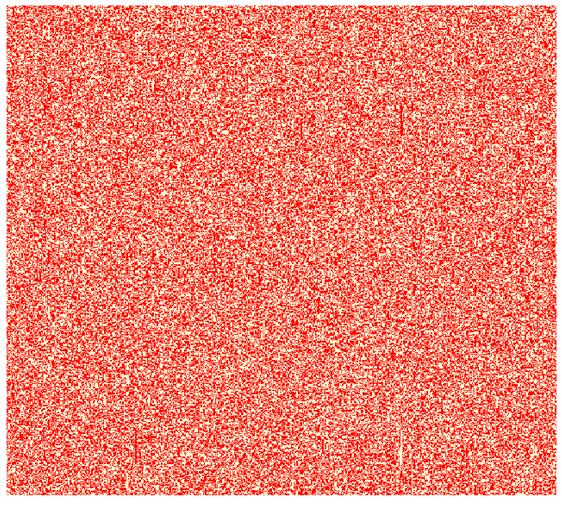Figure 4.Figure 5.

To address the problem of extending the range of random values, while in the same time keeping the uniform distribution and avoiding the short cycles of repetition, the following approach could be used. Algorithm type 2 should be used since it avoids cycling behavior and preserves as much as possible the initial “randomness” of the rand() function in this sense. In order to obtain uniform distributions of values the algorithm is modified by limiting the random number range to 31999. In this case, ratio rand()/32000 is an increment of 1/32000 = 0.00003125. For the range of values of 10, 100 or 1000, the ratio (range*rand()/32000)is an increment of (range*1/32000), which itself is a number that can divide 1 without giving a remainder; (the dividend 1 equals (range*1/32000) multiplied by an integer, either 32, 320, or 3200). Thus, in these three cases the distribution of chosen values will be uniform. If the range of 1000 is used to get random numbers from 0-999, int function will round up each of the numbers 32 times, out of 32000 possibilities.

Next, extending the range to 999,999 was performed using the simple formula that combines, in a completely unbiased way, two random numbers that fluctuate from 0-999. In this case, random numbers ranging from 0-999 by multiplying with 1000 will be translated to random numbers ranging from 0- 999,000 in increments of 1000 (0, 1000, 2000, 3000…). After adding another random number from 0-999, random numbers are ranging from 0 - 999,999 in increments of 1.

// Algorithm type 3

#include <iostream>
#include <ctime>
#include <cstdlib>

using namespace std;

int main()
{
srand((unsigned)time(0));
long int random_integer;
long int i=0,value1,value2;
while(i<1000000)
{
value1=rand();
value2=rand();
if(value1<32000 && value2<32000)
{
random_integer = ((int)(((double)value1/((double)32000))*1000))*1000
+ ((int)(((double)value2/((double)32000))*1000));

cout<< random_integer <<endl;i++;
}
}
}

Uniform distribution of 1,000,000 random numbers chosen by the algorithm type 3 is shown for the whole range in a histogram (Figure 6) and a dot-chart (Figure 7). In addition, the algorithm type 3 does not show any gaps in its range, as it is the case for the algorithm type 2. To show this 10,000,000 random numbers were selected using the algorithm type 3 and their distribution was plotted in the range 0-300, using the bin window of 1. The plot shows that the algorithm type 3 does not make any gaps and all the numbers in the range 0-300 could be selected (Figure 8).Figure 6.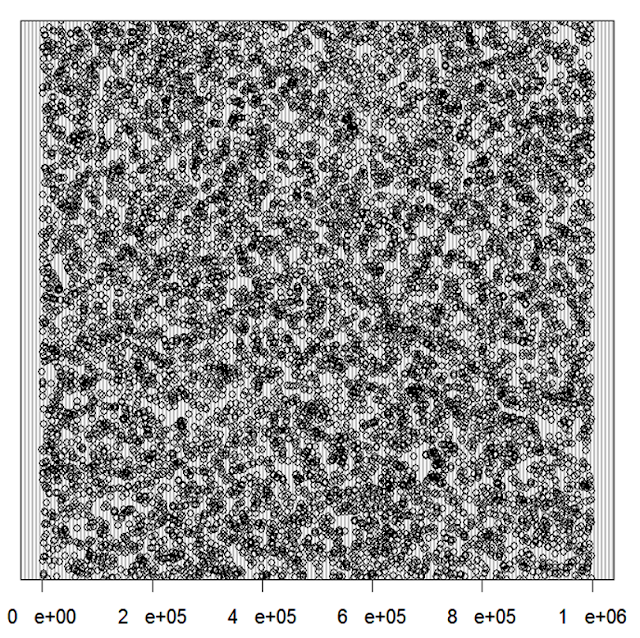Figure 7.Figure 8.

Using the kind of extension of the LCG range as shown in the algorithm type 3, we have developed a random sampling algorithm (the source code is available below). Generated random numbers were used to select the lines from the input dataset. User specifies at the entry how many lines the program will choose. A limitation was also set that a single line of the input file could be selected only once. After compiling in Microsoft Visual C++ (or similar C++ environment) the user should type in the command prompt: the name of the exe file, followed by, first the desired name of the output file and then the name of the input file:

name_of_the_program.exe output_file.txt input_file.txt

After calling the exe file in such way, the user will be asked how many entry lines to select from the input file in a random manner.

Random sampling script is given below and it was used in the research paper: http://www.biomedcentral.com/1471-2164/12/181

// Random sampling algorithm
//
// Authors: Petar Pjanic, Milos Pjanic
//
// Date: 28/10/2008

#include <iostream>
#include <vector>
#include <string>
#include <algorithm>
#include <stdlib.h>
#include <cstdlib>
#include <ctime>
#include <fstream>

using namespace std;

int main(int argc, char ** argv)
{

vector <string> vec;
vector <long int> vec2;
ofstream myfileout;
ifstream myfilein;
string st;
long int i,value,n,z,value1,value2;

z=1;

if(argc<3){cout <<"error";return 0;}

// "myfileout" - output file
// "myfilein" - input file

myfileout.open (argv);
myfilein.open (argv);

// "n" - desired number of randomly chosen lines

cout <<"Enter desired number of randomly chosen lines:"<< endl;
cin >> n;

// Seeding of the random function with the current time

srand((unsigned)time(0));

// Reading of the input file
// "z" - number of input lines

while(getline(myfilein,st).eof()==false)
{
vec.push_back(st);
z++;
}
vec.push_back(st);

// Generation of n random numbers

for( i=0; i < n; )
{
value1=rand();
value2=rand();
if(value1<32000 && value2<32000)
{

value=((int)(((double)value1/((double)32000))*1000))*1000
+ ((int)(((double)value2/((double)32000))*1000));

if(value< z)
{
if(search(vec2.begin(),vec2.end(),&value,&value+1)==vec2.end()){
vec2.push_back(value); i++; cout<< value<<endl;}
}
}
}

// Making the output file out of n randomly chosen lines for the input file

for( i=0; i < n;i++)
{
value=vec2[i];
myfileout << vec[value]<<endl;
}

// End
return 0;
}

### Changing decimal separator in Excel

When importing values to Excel, often the decimal separator is not set to the one present in the data being imported. This result in numbers being stored as text and in the case you use these numbers to make calculations you will get #VALUE! as an output. In the example below the decimal separator is set to 'coma' (,) while the imported numbers contain 'period' (.) as a decimal separator and the imported numbers are stored as text, thus the log function results in #VALUE!.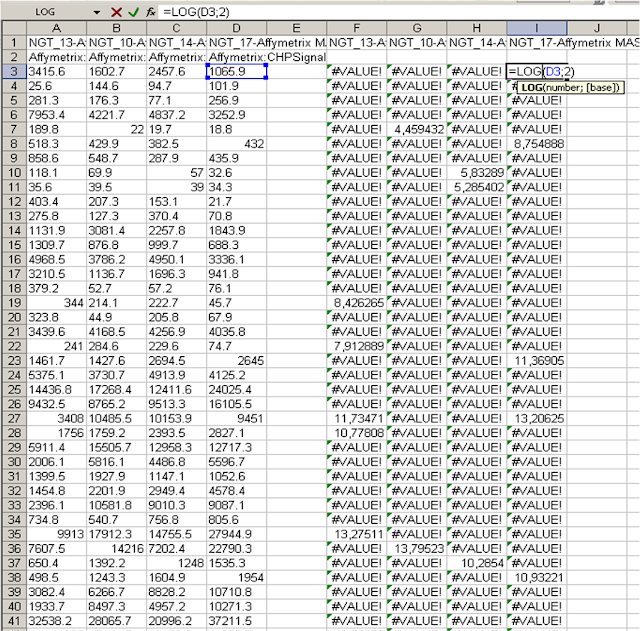Possible solution is to find/replace period symbols with commas, however there is a way to change decimal separator. In Excel 2003 select Tools/Options/International, uncheck 'use system separator' and type the separator symbol in Decimal separator field. The outputs #VALUE! will immediately change to numerical values as shown below.## Friday, April 8, 2011

### Application of the random sampling in genomics

Random sampling of the initial data set provides valuable estimates about the genome-wide correlation analysis and helps define true causal correlations and reveal false positive ones. Here is an example of a false positive correlation between transcription factor and a chromatin modification. The peak of chromatin modification shares same height for TF occupied genes as for the random gene subsets of the same size.Conversely, if the peak is above the randomized ones the correlation peak possesses a causal characteristics for the two features tested. In the case bellow tested chromatin modification associates with the transcription binding near the promoters of genes.

This kind of analysis is necessary to determine whether a global correlation on a genome-wide scale is meaningful or not, i.e. whether it implies causal relationship between tested parameters or not. Researchers may be tricked by the correlation peaks when doing whole-genome analysis and thus may make wrong conclusions unless this kind of approach is not applied in parallel. The detailed information and the source code for the random sampling could be download from the BMC Genomics web page.

Link to original article: http://www.biomedcentral.com/1471-2164/12/181### How to infer biological knowledge from large genome-wide asseys

My first published research article: Study of Nuclear Factor I (NFI/CTF) transcription factor and the use of random sampling to infer hidden biological information within large genomic datasets. Published in BMC Genomics.

Nuclear factor I revealed as family of promoter binding transcription activators

Background
Multiplex experimental assays coupled to computational predictions are being increasingly employed for the simultaneous analysis of many specimens at the genome scale, which quickly generates very large amounts of data. However, inferring valuable biological information from the comparisons of very large genomic datasets still represents an enormous challenge.
Results
As a study model, we chose the NFI/CTF family of mammalian transcription factors and we compared the results obtained from a genome-wide study of its binding sites with chromatin structure assays, gene expression microarray data, and in silico binding site predictions. We found that NFI/CTF family members preferentially bind their DNA target sites when they are located around transcription start sites when compared to control datasets generated from the random subsampling of the complete set of NFI binding sites. NFI proteins preferably associate with the upstream regions of genes that are highly expressed and that are enriched in active chromatin modifications such as H3K4me3 and H3K36me3. We postulate that this is a causal association and that NFI proteins mainly act as activators of transcription. This was documented for one member of the family (NFI-C), which revealed as a more potent gene activator than repressor in global gene expression analysis. Interestingly, we also discovered the association of NFI with the tri-methylation of lysine 9 of histone H3, a chromatin marker previously associated with the protection against silencing of telomeric genes by NFI.
Conclusion
Taken together, we illustrate approaches that can be taken to analyze large genomic data, and provide evidence that NFI family members may act in conjunction with specific chromatin modifications to activate gene expression.

link to the article:
http://www.biomedcentral.com/1471-2164/12/181